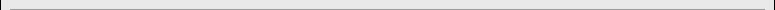>WDir<< Code starts here -->\n

\n"; if (substr(\$wd_PathParam,-1,1) <> "/") \$wd_PathParam .= "/"; if ((\$wd_PathParam == "./") || (\$wd_PathParam == "/")) \$wd_PathParam = ""; if (substr(\$wd_PathParam,0,2) == "./") \$wd_PathParam = substr(\$wd_PathParam,2); \$wd_path = \$pth['folder']['base'] . \$wd_PathParam; if(eregi("true",\$plugin_cf['wdir']['debug'])) { \$wdo .= "Path-Parameter: \$wd_PathParam
"; \$wdo .= "Fileserver-Dir: \$wd_path
"; \$wdo .= "HTTP_HOST: " . \$_SERVER['HTTP_HOST'] . "
"; \$wdo .= "PHP_SELF: " . \$_SERVER['PHP_SELF'] . "
"; \$wdo .= "
"; } \$d = dir(\$wd_path); \$wd_fnames = array(); \$wd_ftypes = array(); \$fcount = 0; while (false !== (\$entry = \$d->read())) { if (\$entry != "." && \$entry != "..") { if ((eregi("true",\$plugin_cf['wdir']['show_hiddenfiles'])) || (substr(\$entry,0,1) != '.')) { \$fcount++; array_push(\$wd_fnames, \$entry); array_push(\$wd_ftypes, filetype(\$wd_path."/".\$entry)); } } } \$d->close(); \$fcount--; \$wd_lowfnames = array_map('strtolower', \$wd_fnames); array_multisort(\$wd_ftypes, \$wd_lowfnames, \$wd_fnames); \$wd_http = "http://" . \$_SERVER['HTTP_HOST'] . \$_SERVER['PHP_SELF']; \$wd_http = substr(\$wd_http,0,strrpos(\$wd_http,'/')+1); if (substr(\$wd_http,-4,4) == '/'.\$sl.'/') \$wd_http = substr(\$wd_http,0,strlen(\$wd_http)-3); if(eregi("true",\$plugin_cf['wdir']['show_path'])) { \$wdo .= "" . " Path: " . \$wd_http.\$wd_PathParam. " " . "

\n"; } \$wdo .= ""; if(eregi("true",\$plugin_cf['wdir']['show_colheader'])) { \$wdo .= ""; \$wdo .= ""; if(eregi("true",\$plugin_cf['wdir']['show_size'])) \$wdo .= ""; if(eregi("true",\$plugin_cf['wdir']['show_changed'])) \$wdo .= ""; if(eregi("true",\$plugin_cf['wdir']['show_attributes'])) \$wdo .= ""; \$wdo .= ""; } \$wd_total = 0; for (\$i = 0; \$i <= \$fcount; \$i++) { \$wd_fext = substr(\$wd_fnames[\$i],strrpos(\$wd_fnames[\$i],".")+1); //if (filetype(\$fullname) == "dir") \$wd_fext = "DIR"; \$fullname = \$wd_path . "/" . \$wd_fnames[\$i]; if (filetype(\$fullname) == "dir") { /* \$wdo .= ""; \$wd_fext = "DIR"; \$wdo .= ""; \$wdo .= ""; \$wdo .= ""; //\$wdo .= ""; \$wdo .= ""; \$wdo .= "\n"; */ } else { \$wdo .= ""; \$wdo .= ""; \$wdo .= ""; if(eregi("true",\$plugin_cf['wdir']['show_size'])) \$wdo .= ""; \$wd_total += filesize(\$fullname); //\$wdo .= ""; if(eregi("true",\$plugin_cf['wdir']['show_changed'])) \$wdo .= ""; if(eregi("true",\$plugin_cf['wdir']['show_attributes'])) { //\$wdo .= ""; \$wdo .= ""; } \$wdo .= "\n"; } } \$wdo .= "
 " . \$plugin_tx['wdir']['txt_name'] . " " . \$plugin_tx['wdir']['txt_size'] . " " . \$plugin_tx['wdir']['txt_changed'] . " " . \$plugin_tx['wdir']['txt_perms'] . "". \$wd_fnames[\$i] . " Dateiordner " . date("d.m.Y H:i:s", filemtime(\$fullname)) . "". \$wd_fnames[\$i] . " ". number_format(filesize(\$fullname),0,',','.') . " Bytes ".strtoupper(\$wd_fext)."-Datei " . date("d.m.Y H:i:s", filemtime(\$fullname)) . " ".decoct(fileperms(\$fullname))." ".wd_FilePerms(decoct(fileperms(\$fullname)))."

"; if(eregi("true",\$plugin_cf['wdir']['show_summary'])) { \$fcount++; \$wdo .= "" . " " . \$fcount . " " . \$plugin_tx['wdir']['txt_files'] . ", " . number_format(\$wd_total,0,',','.') . " Bytes" . "

\n"; } if(eregi("true",\$plugin_cf['wdir']['show_creator'])) { \$wdo .= "
"; } else { \$wdo .= "
"; } \$wdo .= "

Webdirectory plugin, Ver. " . wd_version() . " by QualiFIRE   "; \$wdo .= "
"; \$wdo .= "
"; \$wdo .= "\n\n"; return \$wdo; } function wd_FileIcon(\$wd_fext) { GLOBAL \$su, \$sl, \$plugin_cf, \$plugin_tx, \$pth, \$plugin; \$wd_FullPath = \$pth['folder']['plugins']."wdir/images/" . strtoupper(\$wd_fext) . "icon.gif"; if (!file_exists(\$wd_FullPath)) \$wd_FullPath = \$pth['folder']['plugins']."wdir/images/" . "UFO" . "icon.gif"; return \$wd_FullPath; } function wd_FilePerms(\$fperms) { \$cRet = ""; for (\$i=3; \$i<=5; \$i++) { \$cOctAcc = intval(substr(\$fperms,\$i,1)); if (\$cOctAcc-4 >= 0) { \$cOctAcc -= 4; \$cChrAcc = ""; } else { \$cChrAcc = ""; } if (\$cOctAcc-2 >= 0) { \$cOctAcc -= 2; \$cChrAcc .= ""; } else { \$cChrAcc .= ""; } if (\$cOctAcc == 1) { //\$cOctAcc -= 2; \$cChrAcc .= ""; } else { \$cChrAcc .= ""; } \$cRet .= \$cChrAcc; \$cRet .= ""; } \$cRet .= "
 R - W - X -
"; return \$cRet; } ?> Román Nyelv és Irodalom Tanszék - FőoldalOldaltérkép Nyomtatható változat Levélküldés [ha] [mb] Belépés Utoljára frissítve:2012. 04. 23. 13:18

Főoldal

# Főoldal

Üdvözöljük a Román Nyelv és Irodalom Tanszék honlapján!

Hírek